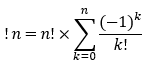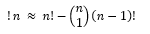# Subfactorial

## Subfactorial: Definition

The subfactorial (!n) calculates the total number of cases n for objects that have each object’s entry not appear in its original location.

The lack of original placement for a set of n objects represents a derangement (Weisstein, 1996)—a permutation of set elements, where no element appears in its original position. At first glance, the written notation for a subfactorial looks to be an incorrectly written factorial. However, the reversal placement of number and exclamation point serves the purpose of solving for specific statistical scenarios.

## Subfactorial Representations

For a n value of 3, the ranked set would contain 3 entries written as {1, 2, 3}. With this set, the current location of the numbers 1 through 3 is called the set’s fixed entries. Finding the total number of arrangements that have no fixed entries is the written understanding of a subfactorial. The written representation of the derangement of numbers for a specific subfactorial of n becomes:

Subfactorial:   !n

For n = 3, the derangements of {1, 2, 3} needs to be found.

The Possible arrangements of the set {1, 2, 3} are: {1, 2, 3} {1, 3, 2} {2, 1, 3} {2, 3, 1} {3, 1, 2} {3, 2, 1}

Where the numbers in red represent the fixed entries that assist in the process of excluding the fixed sets from the deranged sets. The two sets, {2, 3, 1} and {3, 1, 2}, lack the fixed-point property and thus total the subfactorial of 3 to equal 2.

The exact formula for a subfactorial takes the form of a summation:Where n represents all positive integers.

For a numerical representation of the subfactorial concept, one recurrence relationship (Graham, R. L., 1994) acts as a replacement for the solution formula shown above:

!n = n * !(n-1) + (-1)n for n ≥ 1

Given that !3 = 2, finding !4 can be done using the shown relation:

!4 = 4 * !(4-1) + (-4)4 = 4 * !3 + 1 = 4 * 2 + 1 = 9

The recurrence relation is useful in finding the subfactorial value of a positive number. A subfactorial value of a number one less than the number in question needs to be given or solved. That formula was created for a calculation shortcut to the summation formula for time efficiency purposes.

## Proof Explanation

The subfactorial proof for a value of n begins at the factorial concept, n!, which represents all possible arrangements for n objects. From the subfactorial’s definition, only scenarios that have their ranked entries not located at their original placement are counted. A good start to represent the subtraction of all arrangements where at least one entry for  objects is at their original location is written as:The nC1 (inside the first set of parentheses) represents a set that contains at least one fixed point with all other entries being placed elsewhere other than their original location. The (n-1)! attempts to represent all arrangements minus the original ranked set to prevent double counting. However, this approximation has the issue of subtracting arrangements where at least two fixed points are present twice which needs to be added back to the approximation:The nC2 represents sets that contain at least two fixed points with all other entries being placed elsewhere other than their original location. The (n-2)! representing all arrangements minus the original ranked set and the group of sets that have at least one fixed point. The described approach towards accounting for sets that lack fixed point(s) continues this pattern of double subtracting fixed-point sets and the addition of those double-subtracted sets will continue endlessly.

To account for the removal of infinite sets containing fixed points as n goes to infinity, the above two approximations can have their arrangement representations rewritten as:Where k is any positive integer.

Running through the first 5 iterations results in the summation generalization:## Subfactorial Example

Mathematical examples, where the subfactorial is needed, are those that request the total number of scenarios, and the original placement of data (for at least one data entry) is excluded. Consider the following Coffee Buying example:

For one stall at a coffee symposium, their remaining 5 coffee bags were bought up by 5 coffee company representatives. Each coffee bag was made differently and was produced from each of the representative’s companies. If none of the representatives bought a coffee bag that was produced at their company, how many scenarios of this type exist?

Step 1: Set up the subfactorial equation and substitute n for the correct value.Step 2: Evaluate the formula fully to conclude on a total number of derangements.

(5 * 4 * 3 * 2 * 1)(11/30) = 44 scenarios

In conclusion, there are 44 scenarios were each coffee company representative buys a coffee bag that was not produced by their company. Scenario-specific calculations aren’t commonly used outside the realm of statistics. Since cases such as the coffee example have the potential to be useful information for large data analysis teams, it is safe to assume that the subfactorial formula will be picked up from time to time.

## References

R. L. Graham, D. E. Knuth, and O. Patashnik. Concrete Mathematics. AddisonWesley, Reading, MA, USA, 1994, https://www.csie.ntu.edu.tw/~r97002/temp/Concrete%20Mathematics%202e.pdf

Weisstein, Eric E. “Subfactorial.” Concise Encyclopedia of Mathematics, May 1996, archive.lib.msu.edu/crcmath/math/math/s/s826.htm.

CITE THIS AS:
Stephanie Glen. "Subfactorial" From StatisticsHowTo.com: Elementary Statistics for the rest of us! https://www.statisticshowto.com/subfactorial/